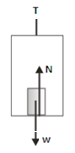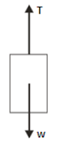# Application of the Newton’s law of the motion in an elevator – problems and solutions

1. A 50-kg person in an elevator. Acceleration due to gravity = 10 m/s2. Determine the normal force exerted on the object by the elevator, if :

(a) the elevator is at rest

(b) the elevator is moving downward at a constant velocity

(c) elevator accelerated upward at a constant acceleration 5 /s2

(d) elevator accelerated downward at a constant 5 m/s2

(e) elevator in a free fall

SolutionKnown :

Person’s mass (m) = 50 kg

Acceleration due to gravity (g) = 10 m/s2

Weight (w) = m g = (50)(10) = 500 Newton

Wanted: The normal force (N)

Solution :

(a) the elevator is at rest

The elevator is at rest so there is no acceleration (a = 0)

We choose the upward direction in the positive direction and the downward direction in the negative direction.

ΣF = m a

N – w = 0

N = w

N = 500 Newton

(b) the elevator is moving downward at a constant velocity

Constant velocity so there is no acceleration (a = 0)

We choose the upward direction in the positive direction and the downward direction in the negative direction.

ΣF = m a

N – w = 0

N = w

N = 500 Newton

(c) elevator accelerated upward at a constant 5 m/s2

The direction of the acceleration is upward, so we choose the positive direction as up.

N – w = m a

N = w + m a

N = 500 + (50)(5)

N = 500 + 250

N = 750 Newton

The person feels the floor pushing up harder than when the elevator is stationary or moving with a constant velocity.

If the person stands on a scale, the scale reads the magnitude of the downward force exerted by the person on the scale. By Newton’s third law, this equals the magnitude of the upward normal force exerted by the scale on the person.

(d) elevator accelerated downward at a constant 5 m/s2

The direction of the acceleration is downward, so we choose the positive direction as down.

w – N = m a

N = w – m a

N = 500 – (50)(5)

N = 500 – 250

N = 250 Newton

The person’s weight is 250 N, less than actual weight w = 500 N.

(e) elevator in a free fall

Free fall means the elevator’s acceleration is the same as the acceleration due to gravity. The magnitude of the acceleration due to gravity is 9,8 m/s2, it’s direction is downward toward the center of the Earth. The speed increases linearly in time by 9,8 m/s during each second.

The direction of the acceleration is downward, so we choose the positive direction as down.

w – N = m a

N = w – m a

N = 500 – (50)(10)

N = 500 – 500

N = 0

[irp]

2. Determine tension in an elevator cable. Elevator’s mass = 2000 kg.

(a) elevator is at rest

(b) elevator accelerated downward at a constant 5 m/s2

(c) elevator accelerated upward at a constant 5 m/s2

(d) elevator in a free fall

Acceleration due to gravity (g) = 10 m/s2

SolutionKnown :

Elevator’s mass (m) = 2000 kg

Acceleration of gravity (g) = 10 m/s2

weight (w) = m g = (2000)(10) = 20,000 Newton

Wanted : The tension force (T)

Solution :

(a) elevator is at rest

elevator is at rest so there is no acceleration (a = 0)

We choose the upward direction as the positive direction and the downward direction as the negative direction.

ΣF = m a

T – w = 0

T = w

T = 20,000 Newton

Tension in cable (T) = elevator’s weight (w) = 20,000 Newton

(b) elevator accelerated downward at a constant 5 m/s2

The direction of the acceleration is downward, so we choose the positive direction as down.

w – T = m a

T = w – m a

T = 20,000 – (2000)(5)

T = 20,000 – 10,000

T = 10,000 Newton

c) elevator accelerated upward at a constant 5 m/s2

The direction of the acceleration is downward, so we choose the positive direction as up.

T – w = m a

T = w + m a

T = 20,000 + (2000)(5)

T = 20,000 + 10,000

T = 30,000 Newton

(d) elevator in a free fall

The direction of the acceleration is downward, so we choose the positive direction as down.

w – T = m a

T = w – m a

T = 20,000 – (2000)(10)

T = 20,000 – 20,000

T = 0

[wpdm_package id=’482′]